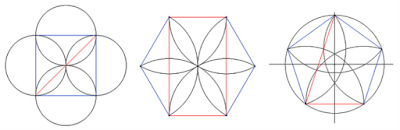## Friday, February 17, 2017

### The Harmony of Being | Geometry in Man, Nature, and CosmosProportional roots: (a) the √2 proportion, (b) the √3 proportion,and (c) the golden mean (Phi) proportion.
Loai M. Dabbour (2012) - Geometry describes the assertions of a mathematical order of the intrinsic nature of the universe. Geometry is the very basis of our reality, and we live in a coherent world governed by underlying laws. Johannes Kepler stated that geometry is underpinning the cosmos, which was based on Plato’s ideas that God created the universe according to a geometric plan. The structure of the universe is determined by and revealed as certain mathematical and geometric constants which represent a conﬁrmation that proportions are the underlying fabric of nature. This can be seen in man, nature, and cosmos.Root proportions based on the square.

By contemplating geometric proportions, an understanding towards the sacred truth can be obtained since geometric proportions are one of the deﬁnitive geometric qualities of life itself. The Holy Quran tells us that man has within himself all what is reﬂected in the universe - the best proportions. Man is the core of God’s creatures; he possesses the most harmonious proportions, reﬂecting of the Divine harmony of being. "We have indeed created man in best of forms" – proportions (Surah At-Tin, 95:4). Leonardo da Vinci illustrated the mathematical proportions of the human body, showing that human being exhibits clearly golden mean proportions in his body based on ratios of 1.618.The Vitruvian Man drawn by Leonardo Da Vinci is based on Vitruvius, who believed that if human proportions could be incorporated into buildings, they would become perfect in their geometry. According to Vitruvius, the distance from ﬁngertip to ﬁngertip should be the same as that from head to toe. The sacred mean rules can be seen in the ratios of body parts throughout the human body. The human body contains in its proportions all the important geometric geodesic measures and functions. The proportions of ideal man are at the center of a circle of invariant cosmic relationships.Proportions of Venus’ and Earth’s mean orbits.
The mathematical harmony of the universe can be seen from the proportions of the planets in our solar system. For example, the ratio of the sacred mean can be seen in the rotations of Venus and Earth around the Sun in that for each ﬁve years that the Earth rotates around the Sun, Venus rotates around it eight times. The connection between 5 and 8, both of which are Fibonacci numbers, is the golden mean proportion (8/5 = 1.6). The result of this motion is that Venus draws a pentagon around the Sun every eight years (Figure A). Figure B shows that a circle is drawn, which represents Venus’ mean orbit. A pentagon is constructed inside it and a small circle placed through the arm-crossing points. The radius of this small circle divides the radius of the large one into golden sections and can be used to space Venus’ orbit from Earth’s orbit. It can be seen from the agreement between eightfold and ﬁvefold geometries that eight touching circles are drawn from Venus’ mean orbit. In turn, the circumference circle is enclosing these eight circles, deﬁning Earth’s mean orbit. The ratio of the mean orbits of Venus’s to Earth is the √2 proportion. The geometric representation of these orbits creates the golden mean proportion.